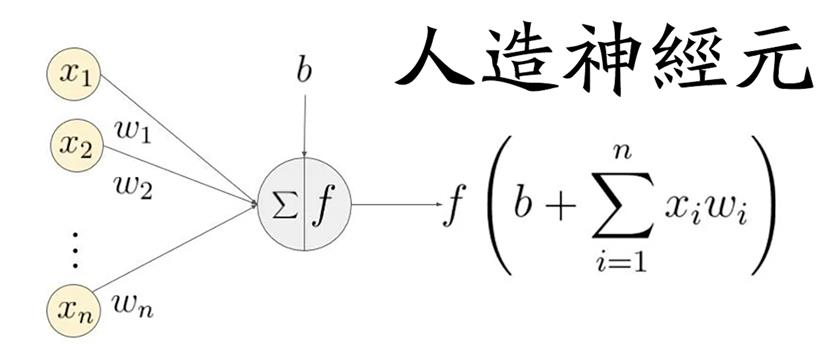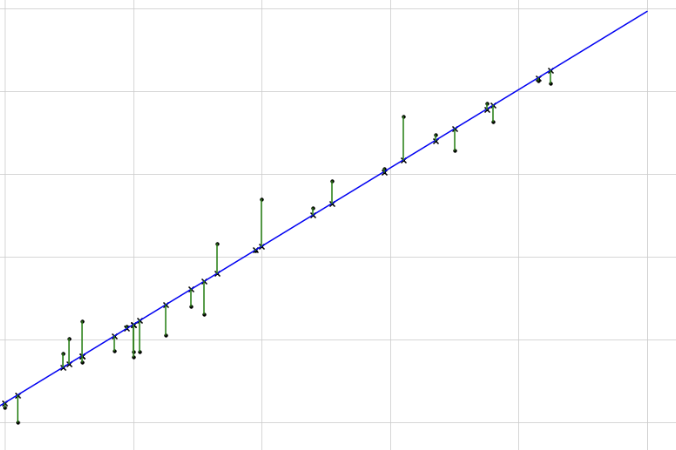• 使用“白话文”来讲解人工智能机器学习的底层逻辑
• 发布于 1周前
• 29 热度
0 评论x代表输入的数据，w代表权重，sum代表求和，b代表偏差，f代表激活函数，最后这个公式运行的结果，就是机器学习的结果。```func neuron(inputs []float64, weights []float64, bias float64) float64 {
if len(inputs) != len(weights) {
panic("inputs and weights must have the same length")
}
sum := bias
for i := 0; i < len(inputs); i++ {
sum += inputs[i] * weights[i]
}
return sum
}```

```inputs := []float64{x1, x2}
weights := []float64{w1, w2}
bias := b
output := neuron(inputs, weights, bias)```

```func backpropagation(inputs []float64, targets []float64, network *Network, learningRate float64) {
// 堆代码 duidaima.com
// 1. 前向传播，计算每个神经元的输出值
outputs := feedforward(inputs, network)

// 2. 计算输出层的误差
outputErrors := make([]float64, len(outputs))
for i := range outputs {
outputErrors[i] = outputs[i] - targets[i]
}

// 3. 反向传播误差，计算每个神经元的误差值
for i := len(network.layers) - 1; i >= 0; i-- {
layer := network.layers[i]
errors := make([]float64, len(layer.neurons))

// 3.1. 计算神经元的误差值
if i == len(network.layers)-1 {
// 输出层的误差
for j := range layer.neurons {
errors[j] = outputErrors[j] * sigmoidPrime(layer.neurons[j].output)
}
} else {
// 隐藏层的误差
for j := range layer.neurons {
errorSum := 0.0
nextLayer := network.layers[i+1]
for k := range nextLayer.neurons {
errorSum += nextLayer.neurons[k].weights[j] * nextLayer.neurons[k].error
}
errors[j] = errorSum * sigmoidPrime(layer.neurons[j].output)
}
}

// 3.2. 将误差值保存到神经元中
for j := range layer.neurons {
layer.neurons[j].error = errors[j]
}
}

// 4. 更新神经网络的权重和偏置
for i := range network.layers {
layer := network.layers[i]

// 4.1. 更新权重
for j := range layer.neurons {
for k := range layer.neurons[j].weights {
if i == 0 {
// 输入层的权重
layer.neurons[j].weights[k] -= learningRate * layer.neurons[j].error * inputs[k]
} else {
// 隐藏层和输出层的权重
prevLayer := network.layers[i-1]
layer.neurons[j].weights[k] -= learningRate * layer.neurons[j].error * prevLayer.neurons[k].output
}
}

// 4.2. 更新偏置
layer.neurons[j].bias -= learningRate * layer.neurons[j].error
}
}
}```